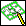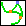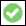1. Click 3D Geometry and then, in the 3D Curve group, click More next toWire Editor.
2. ClickAdjust Curves in the Modify section. The Adjust Curves dialog box opens.
3. Select the first curve.
4. Click the second curve. The two curves must have a common point.
The cyan point is the common point and merge position. The orange points are the fix points of the curves and mark the area of change. It is between these two points that the curves are modified.
5. Select which curve(s) should be modified: Both or Second.
6. Select the Tangential or curvature continuous condition at the Fix Points: Tangential or Curv Cont (curvature continuous).
7. Set the following options:
Merge Curves: Merges both curves into one curve. The second curve is deleted and the first curve is modified. If Merge Curves is off, both curves are modified. If two curves are already adjusted, they can be merged.
Through Common Pt: Adjusts the curves at their common point. When switched on, the new adjusted curve goes through the common point.
8. Clickto complete the operation.
Limitations
The creation and modification of closed, non-periodic spline curves is not allowed.
You cannot delete points from
a closed curve with 3 points.
an open curve with 2 points.
Analytic geometry such as straight lines, arcs, etc. is converted into spline curves.
Adjust does not work for closed curves because there is no end point to change.
Only 3D curves in wire parts can be used.
The first curve (Curve 1) can be an edge from a solid or face part. If so, then only the Second side option in Modify Curves is available.